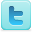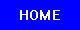﻿ Lagrange's Four-Square Theorem: Lesson Plans, Proofs, Articles and Background Information
Lagrange's Four-Square Theorem
Lesson Plans, Proofs, Articles and Background Information

Resources

### Definition

Lagrange's four-square theorem: Every non-negative integer can be expressed as the sum of four squares.

For example:

9 = 12 + 22 + 22 + 02
36 = 52 + 32 + 12 + 12

### Background Information

• Lagrange's four-square theorem - Wikipedia [View Resource]
• Lagrange's Four-Square Theorem - MathWorld [View Resource]
• Adrien-Marie Legendre Life and Work [View Resource]

### K-12 Lesson Plans, Proofs and Science Fair Projects

• Lagrange's four-square theorem proof [View Resource]
• An applet decomposing numbers as sums of four squares [View Resource]
• A New Method to Prove Euler's Equation by Using the Lagrange Mean Value Theorem[View Resource]
• Lagrange Four Square Theorem (Bachet Conjecture) Calculation ind Instructions [View Resource]
• Lagrange's Four Square Theorem Proof [View Resource]

### Undergraduate Lesson Plans, Proofs, Studies and Articles

• Representations of binary forms by quinary quadratic forms [View Resource]
• Lagrange's four-square theorem proof using convex geometry [View Resource]
• A Proof of Lagrange's Four Square Theorem Using Quaternion Algebras [View Resource]
• Cosets and Cardinality Lesson [View Resource]
• Patterns in Prime Numbers: The Quadratic Reciprocity Law [View Resource]

### Theses and Dissertations

• Convex functions and optimization techiniques [View Resource]
• Automatic Formulation of Lagrangian DAEs [View Resource]